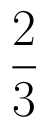Select Page

# CBSE MCQ in English Answers for Maths 12 Science Matrices

CBSE MCQ in English Answers for Maths 12 Science Matrices to enable students to get Answers in a narrative video format for the specific question.

Expert Teacher provides CBSE MCQ Answers for Maths 12 Science Matrices through Video Answers in English language. This video solution will be useful for students to understand how to write an answer in exam in order to score more marks. This teacher uses a narrative style for a question from Matrices not only to explain the proper method of answering question, but deriving right answer too.

Please find the question below and view the Answer in a narrative video format.

Question:

## Similar Questions from CBSE, 12th Science, Maths, Matrices

Question 1 : Find the value of X, ifand. (View Answer Video)

Question 2 : Using elementary transformation, find the inverse of the matrix. (View Answer Video)

Question 3 : Find the value of z,  from the equation:(View Answer Video)

Question 4 : Compute:. (View Answer Video)

Question 5 : If,find the value of y.  (View Answer Video)

### Determinant

Question 1 :  If A is a square matrix of order 3 and |A| = 5 then |3A| = ? (View Answer Video)

Question 2 : Find the equation of the line joining (1, 2) and (3, 6) using determinants. (View Answer Video)

Question 3 : Find the adjoint  of the matrix. (View Answer Video)

Question 4 : Let A be a non-singular square matrix of order 3 * 3. Then | adj A | is equal to: (View Answer Video)

Question 5 : Evaluate the determinant:. (View Answer Video)

### Probability

Question 1 : An insurance company insured 2,000 cyclists, 4,000 scooter drivers and 6,000 motorbike drivers. The probability of an accident involving a cyclist, scooter driver and a motorbike driver are 0.01, 0.03, and 0.15 respectively. One of the insured persons meets with an accident. What is the probability that he is a scooter driver?     (View Answer Video)

Question 2 : A problem in mathematics is given to 4 students A, B, C, D. Their chances of solving the problem, respectively, areand. What is the probability that atmost one of them solve the problem?  (View Answer Video)

Question 3 :  Four cards are drawn successively with replacement from a well shuffled deck of 52 cards. What is the probability that:
All the four cards are spades?

Question 4 : If E and F are two events such that,, Find P(not E and not F). (View Answer Video)

Question 5 : An urn contains 4 balls. Two balls are drawn at random from the urn (without replacement) and are found to be white. What is the probability that all the four balls in the urn are white?  (View Answer Video)

### Three Dimensional Geometry

Question 1 : Find the distance of the point (1, -2, 3) from the plane x-y+z=5 measured parallel to the line(View Answer Video)

Question 2 : Find the distance between the point (-1, -5, -10) and the point of intersection of lineand plane
Question 3 : Find the equation of plane passing through the line of intersection of the planesandand passing through the point (3, -2, -1). Also, find the angle between the two given planes. (View Answer Video)